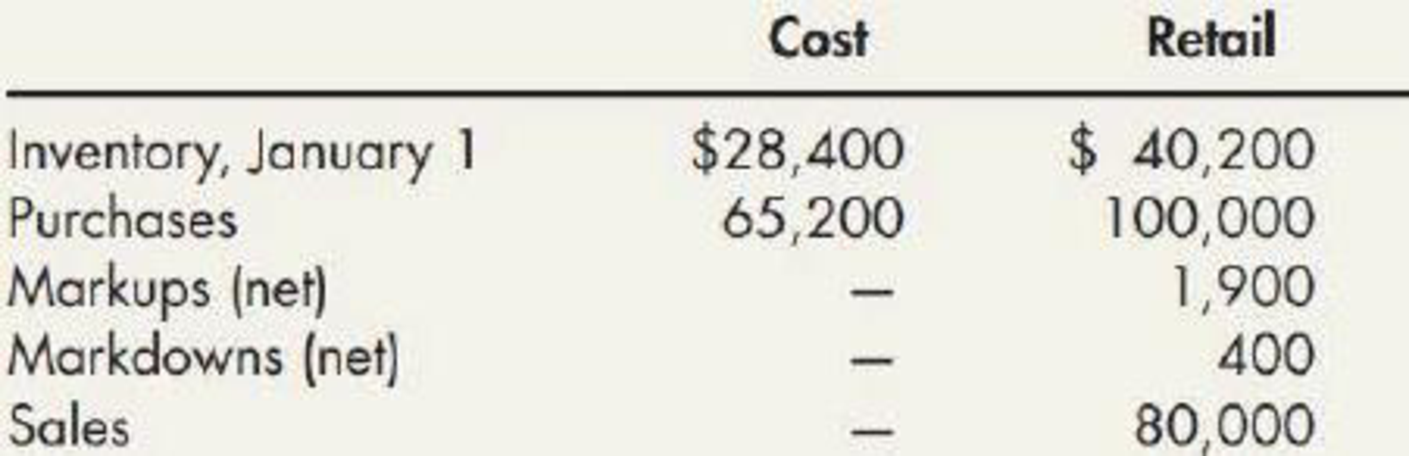Chapter 8, Problem 11E### Intermediate Accounting: Reporting...

3rd Edition
James M. Wahlen + 2 others
ISBN: 9781337788281

#### Solutions

Chapter
Section### Intermediate Accounting: Reporting...

3rd Edition
James M. Wahlen + 2 others
ISBN: 9781337788281
Textbook Problem
41 views

# Retail Inventory Method Harmes Company is a clothing store that uses the retail inventory method. The following information relates to its operations during the year:Required:Compute the ending inventory by the retail inventory method for the following cost flow assumptions (round the cost-to-retail ratio to 3 decimal places): 1. FIFO 2. average cost 3. LIFO 4. lower of cost or market (based on average cost)

1.

To determine

Calculate the cost of ending inventory by the retail method using FIFO cost flow.

Explanation

Retail inventory method: It takes into account all the retail amounts that is, the current selling prices. Under this method, the goods available for sale, at retail is deducted from the sales, at retail to determine the ending inventory, at retail.

Conventional Retail Method: Conventional retail method refers to the estimation of the lower of average cost or market by eliminating the markdowns from the calculation of the cost-to-retail percentage.

In this case, the cost-to-retail percentage will be determined by dividing the goods available for sale at cost by the goods available for at retail (excluding markdowns). Thus, the conventional retail method will always result in lower estimation of ending inventory when the markdowns exist.

FIFO: Under this inventory method, the units that are purchased first are sold first. Thus, it starts from the selling of the beginning inventory, followed by the units purchased in a chronological order of their purchases took place during a particular period.

Calculate the cost of ending inventory by the retail method using FIFO cost flow:

 Ending Inventory - FIFO Details Cost ($) Retail ($) Purchases 65,200 100,000 Markups 1,900 Markdowns 0 (400) 65,200 101,500 Add: Beginning inventory 28,400 40,200 Goods available for sale 93,600 141,700 Less: Sales (80,000) Ending inventory at retail $61,700 Ending inventory at cost$39,611

Table (1)

Working note 1:

Calculate ending inventory at cost:

Step 1: Calculate cost-to-retail ratio...

2.

To determine

Calculate the cost of ending inventory by the retail method using average cost flow.

3.

To determine

Calculate the cost of ending inventory by the retail method using LIFO cost flow.

4.

To determine

Calculate the cost of ending inventory by the retail method using lower of cost or market rule.

### Still sussing out bartleby?

Check out a sample textbook solution.

See a sample solution

#### The Solution to Your Study Problems

Bartleby provides explanations to thousands of textbook problems written by our experts, many with advanced degrees!

Get Started